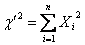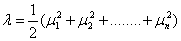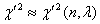# Non Central Chi Square distribution Assignment Help

## 11.11 Non Central Chi Square distribution:

The chi-square distribution defined as the sum of the squares of independent standard normal variates is often referred to as the central chi-square distribution. The distribution of the sum of the squares of independent normal variates each having unit variance but with possibly non zero means is known as non-central chi-square distribution. Thus if Xi, (i=1,2,…,n)are independent N(μi, 1), random variables thenHas the non central chi-square distribution with n degrees of freedom. Intuitively, this distribution would seem to depend upon the n parameters μ1, μ2,…….., μn but it will be seen that it depends on these parameters only through the non-centrality parameter.## Non Central Chi Square distribution Assignment Help By Online Tutoring and Guided Sessions at AssignmentHelp.Net

And we write,.